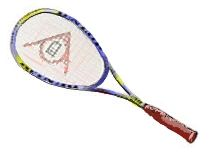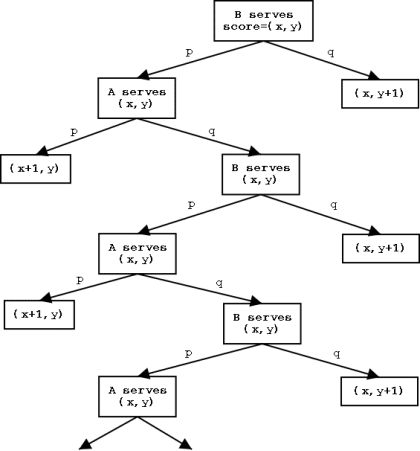# Playing Squash

##### Age 16 to 18

Published 2001 Revised 2008In the game of squash, players gain "points'' by winning "rallies''. If the "server'' wins a rally, he or she wins a point; otherwise the service changes hands with no points gained. In theory the game could go on for ever with each player losing all their serves. Note that if in two consecutive rallies both players lose their serve, then the situation is exactly the same as it was before these two serves.

Normally the game is won by the first player to reach 9 points, typically by 2 or more points. But if the score reaches 8-8 then the person due to receive serve can call "9'' (in which case the first to reach 9 wins) or call "10'' (in which case the first to reach 10 wins). In December 2000 the problem was set of deciding whether a player should call "9'' or "10'' if that player has a fixed probability of winning any particular rally, regardless of whether serving or receiving.

We call the players A and B, and we assume that the probability that A wins a rally is $p$, whether he serves or not, and whatever the score is. The corresponding probability for B is $q$, where $p+q=1$.

At any given time there are four outcomes to be considered, namely A or B is the first to serve, and A or B wins the next point. There may be many rallies before anyone scores this point We are interested in finding the four probabilities:
\begin{equation*} Pr(\hbox{A wins the next point given that A serves first})= \theta, \end{equation*} \begin{equation*} Pr(\hbox{A wins the next point given that B serves first})= \varphi, \end{equation*} \begin{equation*} Pr(\hbox{B wins the next point given that A serves first})= \lambda, \end{equation*} \begin{equation*} Pr(\hbox{B wins the next point given that B serves first})= \mu. \end{equation*}
Let us find $\theta$. One possibility is that $A$ wins the first rally, and hence the first point; he does this with probability $p$. If not, he loses it with probability $q$ and $B$ then serves. Then for $A$ to win the next point he must win the next rally (with probability $p$) and then the situation returns to that in which $A$ serves and wins the next point with probability $\theta$. Thus $\theta = p + qp\theta$, and hence $Pr(\hbox{A wins the next point given that A serves first})= \theta = {p\over 1-pq}.$ Next, we find $\varphi$. As $B$ serves first, $A$ must win the next rally (with probability $p$). The position now is that $A$ is serving and must win the next point (which he does with probability $\theta$). Thus $\varphi = p\theta$, and hence $Pr(\hbox{A wins the next point given that B serves first})= \varphi ={p^2\over 1-pq}.$ By interchanging $A$ with $B$, and $p$ with $q$, we see that $Pr(\hbox{B wins the next point given that A serves first})= \lambda ={q^2\over 1-pq},$ and $Pr(\hbox{B wins the next point given that B serves first})= \mu ={q\over 1-pq}.$ Note that $Pr(\hbox{A or B wins the next point given that B serves first})= \varphi + \mu,$ and $\varphi + \mu = {p^2\over 1-pq}+ {q\over 1-pq} ={p(1-q)+q\over 1-pq}={p+q-pq\over 1-pq}={1-pq\over 1-pq}=1.$ It follows that if $B$ serves first, then the probability that $A$ or $B$ eventually wins a point is one; hence the probability that the game goes on for ever is zero! You may like to draw a tree diagram to illustrate this, and you will find that a succession of rallies in which nobody scores a point is represented by a long 'zig-zag' in your tree diagram. The start of the tree diagram is given below.The rules of squash say that if the position is reached when the score is $(8,8)$ (we give A's score first), and $B$ is to serve, then $A$ must choose between the game ending when the first player reaches $9$ or when the first player reaches $10$. We want to decide what is the best choice for $A$ to make (when $p$ and $q$ are known).

If $A$ chooses $9$, then he wins the game with probability $\varphi$. Suppose now that $A$ chooses $10$; then the sequence of possible scores and their associated probabilities are as follows:

Sequence of scores Probability Probability in terms of $p,q$
$(8,8) \to (9,8) \to (10,8)$ $\varphi \times \theta$ $p^3/(1-pq)^2$
$(8,8)\to (8,9)\to (9,9) \to (10,9)$ $\mu\times\varphi\times\theta$ $p^3q/(1-pq)^3$
$(8,8) \to (9,8) \to (9,9) \to (10,9)$ $\varphi \times \lambda\times \varphi$ $p^4q^2/(1-pq)^3$

It follows that the choice of $9$ by $A$ is best for $A$ if $\varphi > \varphi\theta + \mu\varphi\theta + \varphi^2\lambda$, or, equivalently, $1 > \theta + \mu\theta + \varphi\lambda$. In terms of $p$ and $q$ this inequality is $1 > {p\over 1-pq} + {pq\over (1-pq)^2} + {p^2q^2\over (1-pq)^2},$ and this is equivalent to $(1-pq)^2 > p(1-pq)+pq+p^2q^2.$ After simplification (remember that $p+q=1$), this is equivalent to $p^2-3p+1> 0$. This means that $A$ should choose $9$ if $p < 0.38$ and should choose $10$ if $p> 0.38$.

[See the problem Squash on the NRICH site.]